## EdgeCollapsableMesh

### Definition

The concept EdgeCollapsableMesh describes the requirements for the type of triangulated surface mesh that can be passed to the simplification algorithm.

The surface must be structurally equivalent to a polyhedral surface having only triangular faces. It can have any number of connected components, boundaries (borders and holes) and handles (arbitrary genus).

HalfedgeGraph

### Valid Expressions

The mesh simplification algorithm requires the free function collapse_triangulation_edge.

template<class EdgeCollapsableMesh>
typename boost::graph_traits<EdgeCollapsableMesh>::vertex_descriptor
 halfedge_collapse ( typename boost::graph_traits::edge_descriptor const& ue, EdgeCollapsableMesh& mesh)
Collapses the undirected edge (v0v1,v1v0) replacing it with v0 or v1, as described in the following paragraph.
 Precondition: This function requires mesh to be an oriented 2-manifold with or without boundaries. Furthermore, the undirected edge (v0v1,v1v0) must satisfy the link condition [DEGN99], which guarantees that the surface is also 2-manifold after the edge collapse.

Let v0 be the source and v1 be the target vertices of v0v1.

For e { v0v1,v1v0 }, let en and ep be the next and previous edges, that is en = next_edge(e, mesh), ep = prev_edge(e,mesh), and let eno and epo be their opposite edges, that is eno = opposite_edge(en, mesh) and epo = opposite_edge(ep,mesh).

Then, after the collapse of (v0v1,v1v0) the following holds:

• The edge e is no longer in mesh.
• One of {v0,v1} is no longer in mesh while the other remains. 1 Let vgone be the removed vertex and vkept be the remaining vertex.
• If e was a border edge, that is get(is_border, e, mesh) == true, then next_edge(ep) == en, and prev_edge(en) == ep.
• If e was not a border edge, that is get(is_border, e, mesh) == false, then ep and epo are no longer in mesh while en and eno are kept in mesh.
• For all edges ie in in_edges(vgone,mesh), target(ie,mesh) == vkept and source(opposite_edge(ie),mesh) == vkept.
• No other incidence information has changed in mesh.

The function returns vertex vkept (which can be either v0 or v1).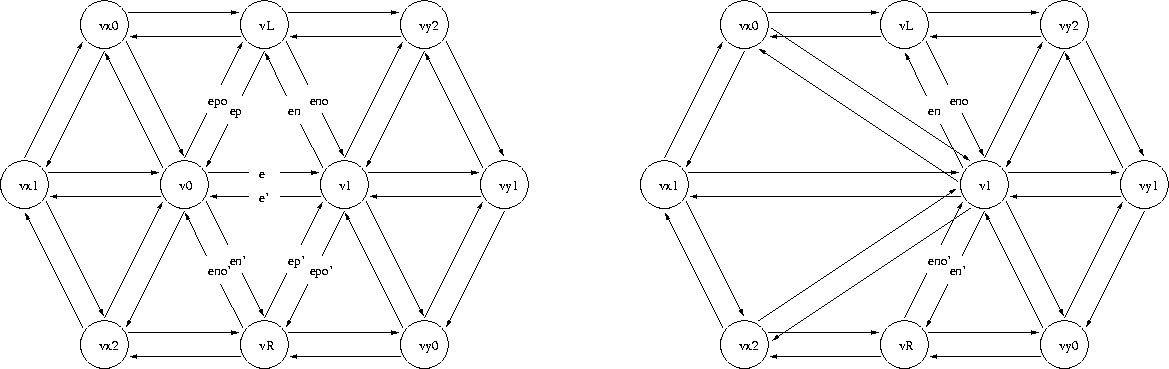Figure 49.1:  General case. The following mesh elements are removed: triangles (v0,v1,vL) and (v1,v0,vR), edges (e,e'), (ep,epo) and (ep',epo'), and vertex v0.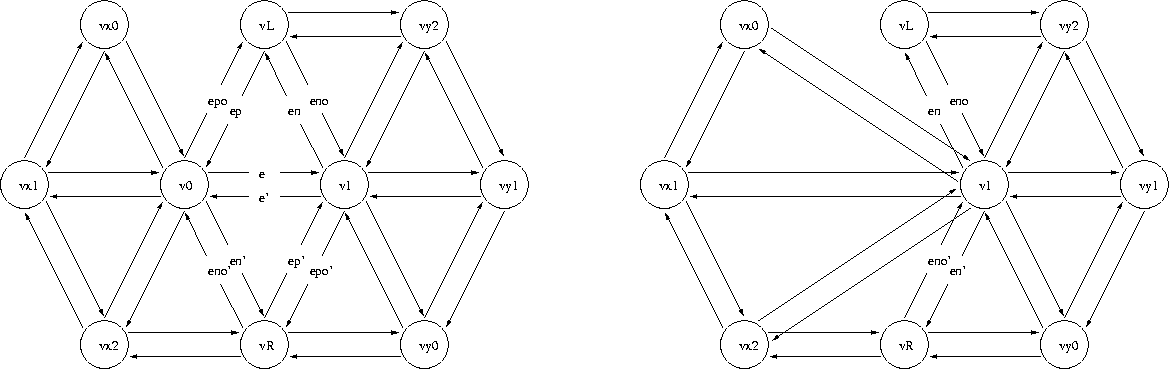Figure 49.2:  When the collapsing edge is not itself a border, but is incident upon a border edge that is removed, the operation is the same as in the general case.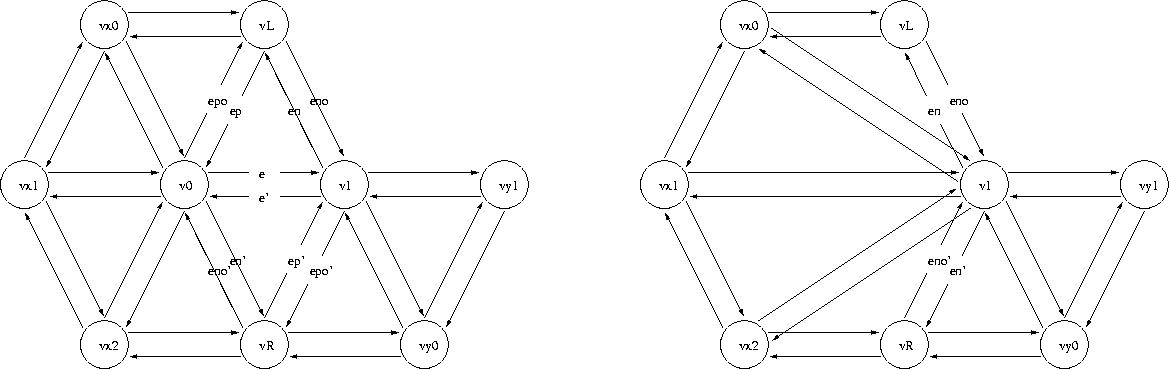Figure 49.3:  When the collapsing edge is not itself a border, but is incident upon a border edge that is not removed, the operation is still the same as in the general case.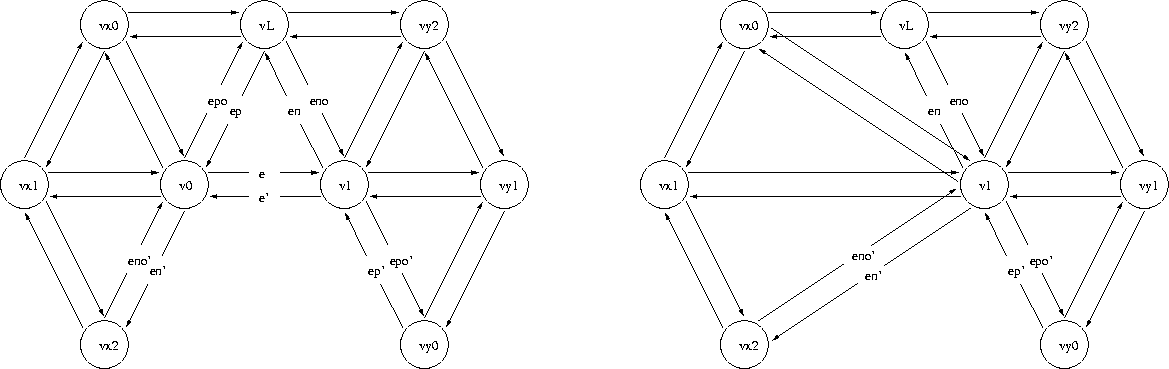Figure 49.4:  When the collapsing edge is itself a border, only 1 triangle is removed. Thus, even if (ep',epo') exists, it's not removed.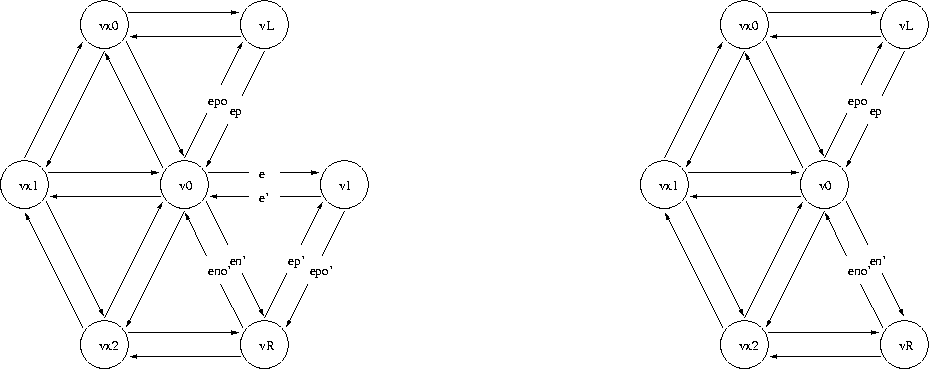Figure 49.5:  This figure illustrates the single exceptional case when removing (v0,v1) neccesarily implies removing (v1), thus (v0) remains.

### Has Models

CGAL::Polyhedron_3<Traits>
(If it has only triangular faces, and via External Adaptation, which is described in [SLL02] and this Bgl web page: http://www.boost.org/libs/graph/doc/leda_conversion.html).